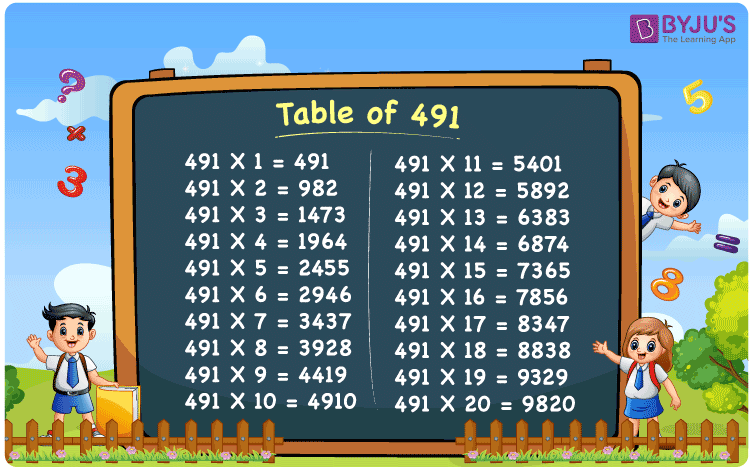Checkout JEE MAINS 2022 Question Paper Analysis : Checkout JEE MAINS 2022 Question Paper Analysis :

# Table of 491

The table of 491 is the repeated addition of 491 as many times as required to get the values of multiplication of 491 by integers. That means to get the value of multiplication of 491 by 3; we must add 491 thrice, i.e. three times. Thus, 491 × 3 = 1473 or 491 + 491 + 491 = 1473. In this way, we can write the math table of 491 up to n number of times. In this article, you can access the chart, table, and PDF of the 491 times table.

## Table of 491 Chart## What is the 491 Times Table?

Let’s have a look at the 491 times table given below using multiplication and repeated addition. This will help you to learn how to create the table of 491 up to 10 times.

 Using multiplication Using repeated addition 491 × 1 = 491 491 491 × 2 = 982 491 + 491 = 982 491 × 3 = 1473 491 + 491 + 491 = 1473 491 × 4 = 1964 491 + 491 + 491 + 491 = 1964 491 × 5 = 2455 491 + 491 + 491 + 491 + 491 = 2455 491 × 6 = 2946 491 + 491 + 491 + 491 + 491 + 491 = 2946 491 × 7 = 3437 491 + 491 + 491 + 491 + 491 + 491 + 491 = 3437 491 × 8 = 3928 491 + 491 + 491 + 491 + 491 + 491 + 491 + 491 = 3928 491 × 9 = 4419 491 + 491 + 491 + 491 + 491 + 491 + 491 + 491 + 491 = 4419 491 × 10 = 4910 491 + 491 + 491 + 491 + 491 + 491 + 491 + 491 + 491 + 491 = 4910

## Multiplication Table of 491

The multiplication table of 491 contains the multiples of 491 as shown in the table given below.

 491 × 1 = 491 491 × 2 = 982 491 × 3 = 1473 491 × 4 = 1964 491 × 5 = 2455 491 × 6 = 2946 491 × 7 = 3437 491 × 8 = 3928 491 × 9 = 4419 491 × 10 = 4910 491 × 11 = 5401 491 × 12 = 5892 491 × 13 = 6383 491 × 14 = 6874 491 × 15 = 7365 491 × 16 = 7856 491 × 17 = 8347 491 × 18 = 8838 491 × 19 = 9329 491 × 20 = 9820

## Example on the Table of 491

Question:

The cost of a kid’s wooden xylophone is Rs. 491. What is the price of 7 such xylophones?

Solution:

The cost of 1 xylophone = Rs. 491

Number of xylophones = 7

Total amount = ?

Using the table of 491, 491 × 7 = 3437.

Therefore, the total cost of 7 xylophones = Rs. 491 × 7 = Rs. 3437.

## Frequently Asked Questions on Table of 491

### What is the multiplication table of 491?

The multiplication table of 491 is the product of 491 and the consecutive natural numbers. Some of the entries of the 491 times table are given below:
491 × 1 = 491
491 × 2 = 982
491 × 3 = 1473
491 × 4 = 1964

### What is 491 multiplied by 15?

491 multiplied by 15 is equal to 7365, i.e. 491 × 15 = 7365.

### 491 times of which number is equal to 9329?

491 times 19 is equal to 9329, i.e. 491 × 19 = 9329.